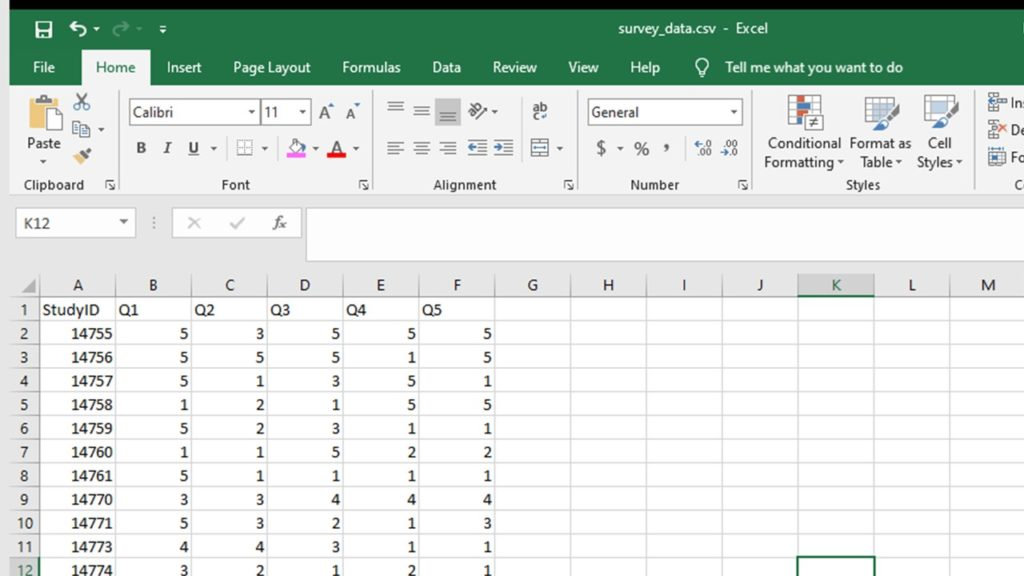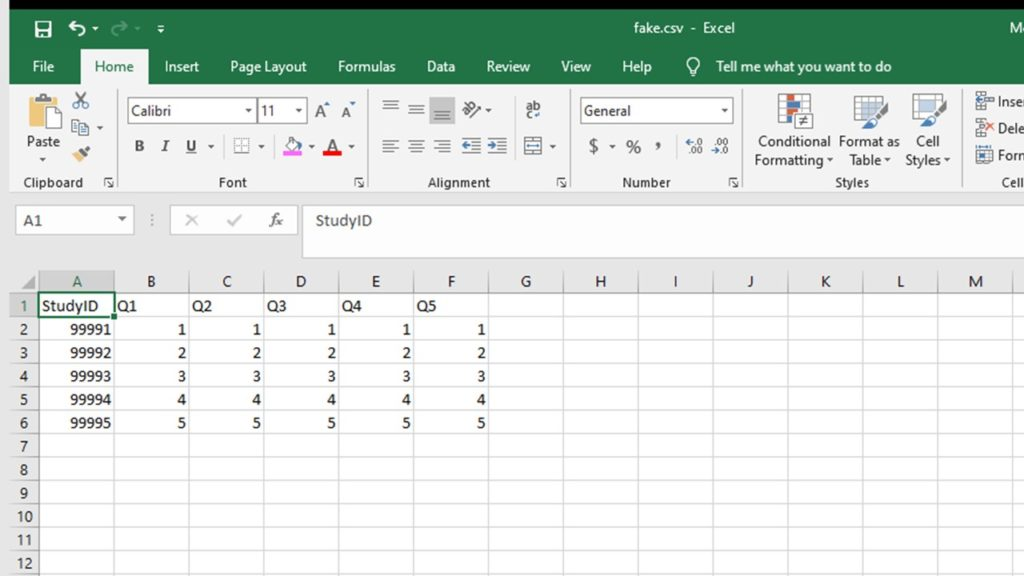# Doing Surveys? Try my R Likert Plot Data Hack!

Like many R packages, the Likert package in R is awesome, but it is particular about how you make you Likert plot data before you put it in the plot. I’ve gotten it down to nine steps.

## Using Features of Likert Plot Data to Improve Plot Appearance

• The first six steps are a workaround to get it so that you can use the levels in factor variables in the Likert plot data as labels in the legend without getting any data errors.
• Steps seven and eight are to make it so the plot prints the full original Likert statements next to the bar by copying them the actual names of dataframe columns.
• The final step shows how I like to formulate my Likert plot code with options that uses the Likert plot data we created.

`survey1 <- read.csv("survey_data.csv", header = TRUE, sep = ",")````StudyID <- c("99991", "99992", "99993", "99994", "99995")
Q2 <- c(1, 2, 3, 4, 5)
Q3 <- c(1, 2, 3, 4, 5)
Q4 <- c(1, 2, 3, 4, 5)
Q5 <- c(1, 2, 3, 4, 5)
fake <- data.frame(StudyID, Q1, Q2, Q3, Q4, Q5)
``````survey2 <- rbind(survey1, fake)
survey2\$Q1_f <- as.factor(survey2\$Q1)
survey2\$Q2_f <- as.factor(survey2\$Q2)
survey2\$Q3_f <- as.factor(survey2\$Q3)
survey2\$Q4_f <- as.factor(survey2\$Q4)
survey2\$Q5_f <- as.factor(survey2\$Q5)
```
```factor_levels <- c("Strongly Disagree","Somewhat Disagree","Neither Agree nor Disagree",
"Somewhat Agree","Strongly Agree")

levels(survey2\$Q1_f) <- factor_levels
levels(survey2\$Q2_f) <- factor_levels
levels(survey2\$Q3_f) <- factor_levels
levels(survey2\$Q4_f) <- factor_levels
levels(survey2\$Q5_f) <- factor_levels
```
```nrow(survey2)
survey3 <- subset(survey2, StudyID < 99991)
nrow(survey3)
```
```colnames(survey3)
survey4 <- survey3[,7:11]
colnames(survey4)
```
```VarHeadings <- c("I want to live in a world with unicorns.",
"Whenever given a choice, I choose chocolate.","My hair is too long.",
"There really aren't any reasons to do cross-stitch.","Rats are misunderstood.")

colnames(survey4)
```
```library(likert)
p <- likert(survey4)
a <- likert.bar.plot(p, legend.position = "right", text.size = 4) +
theme(text = element_text(size = rel(4)),axis.text.y = element_text(size = rel(2))) +
theme_update(legend.text = element_text(size = rel(0.7))) +
theme_classic()
plot(a)
```

Updated October 10, 2020. Added FTC disclaimer and other edits on December 5, 2020. Formatting edits September 12, 2021 and October 11, 2022. Added banners March 6, 2023.

I love the Likert package in R, and use it often to visualize data. The problem is that sometimes, I have sparse data, and this can cause problems with the package. This blog post shows you a workaround, and also, a way to format the final plot that I think looks really great!

### 2 thoughts on “Doing Surveys? Try my R Likert Plot Data Hack!”

1.VJ Johnson says:

This was excellent! Thank you for being my collaborator. I really wanted to use this package and was getting so frustrated with how to make it work; adding in the fake data did the trick 🙂

2.Monika Wahi says:

Awesome! If you go to the video, you will see one of the commenters wrote some more complex code to solve the problem. I’m not smart enough for that – so adding fake data does the trick!

This site uses Akismet to reduce spam. Learn how your comment data is processed.